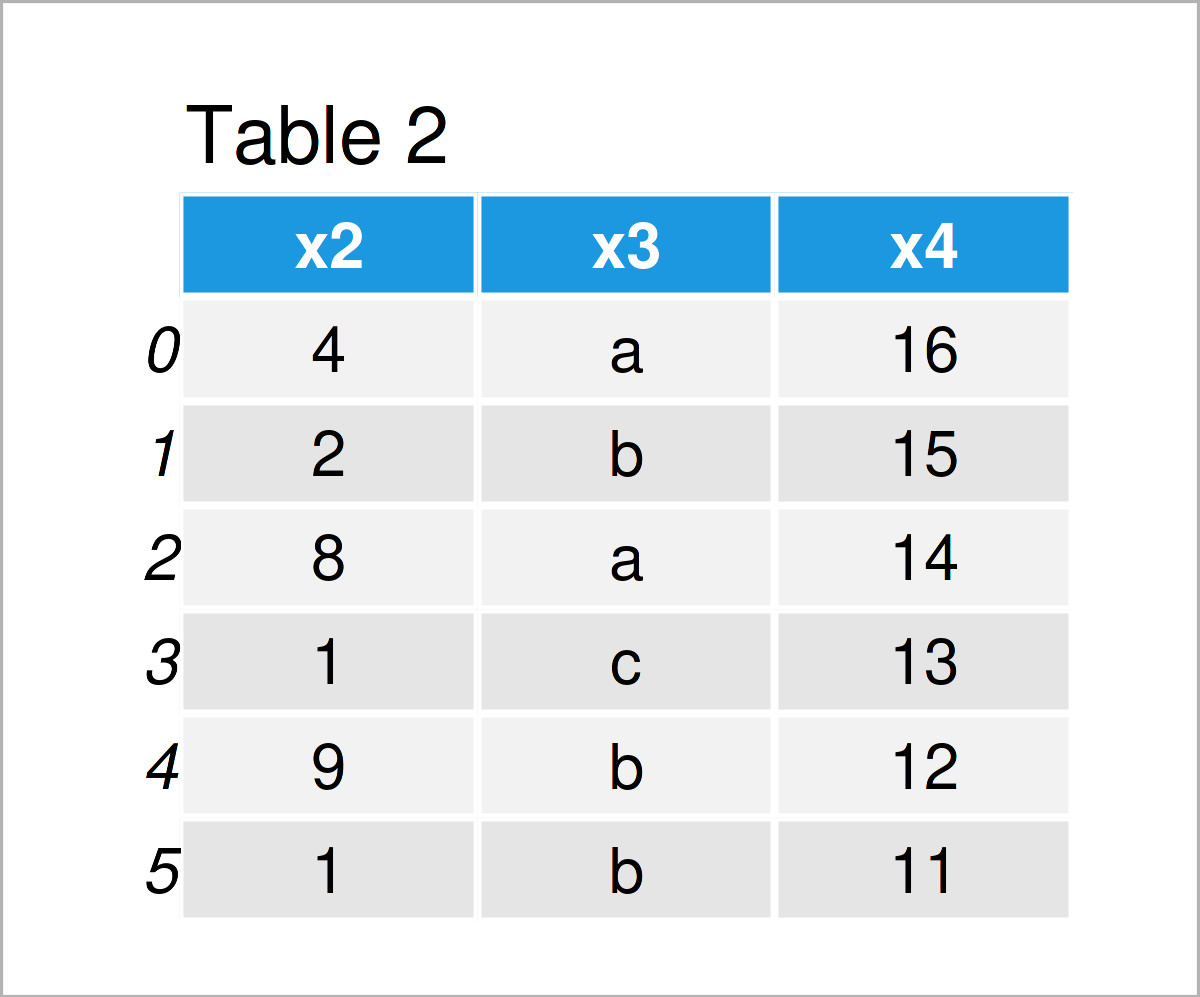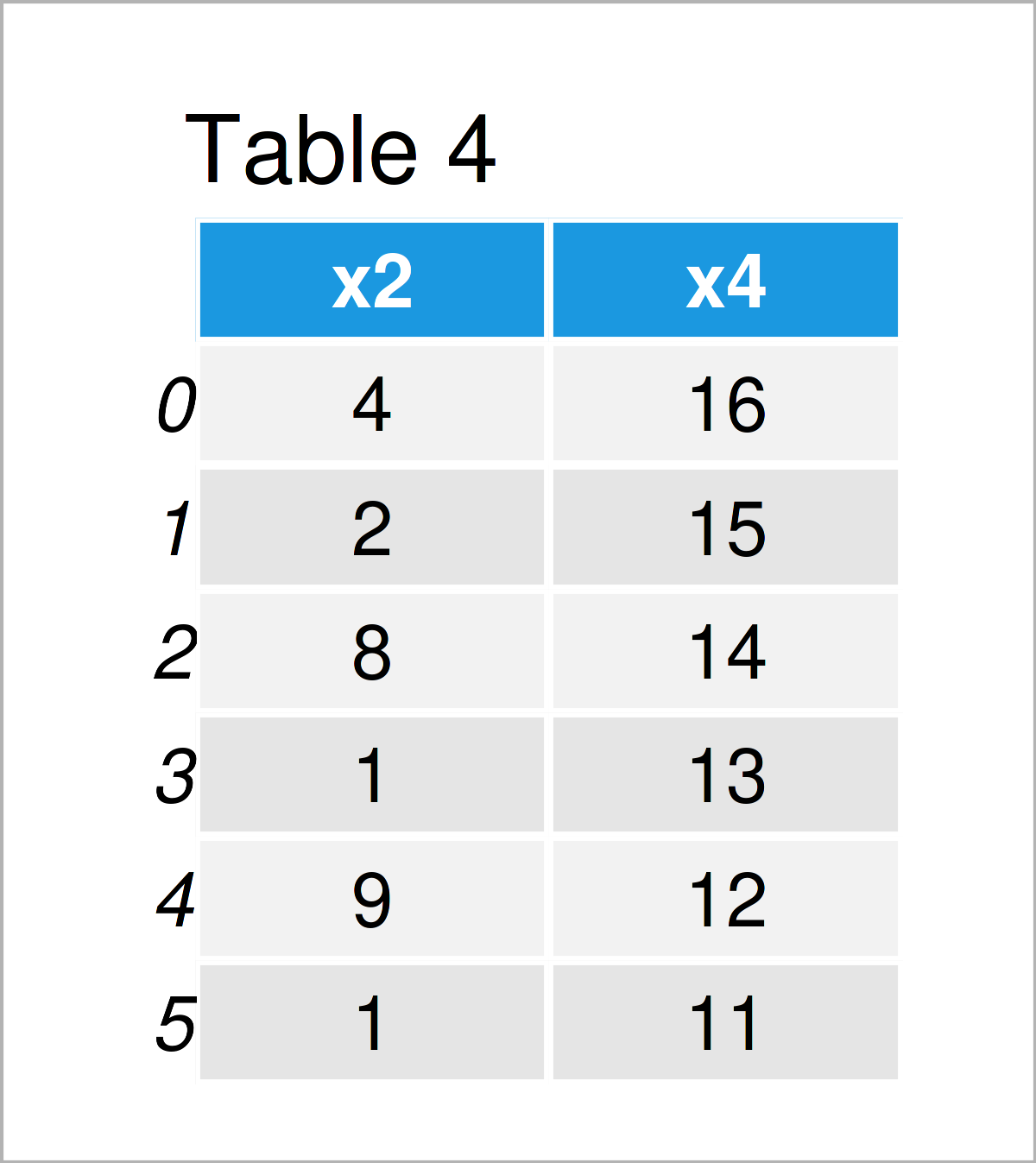# Delete Column of pandas DataFrame in Python (3 Examples)

This article shows how to drop one or multiple variables from a pandas DataFrame in Python programming.

The tutorial will contain these content blocks:

Let’s dig in:

## Example Data & Libraries

In order to use the functions of the pandas library, we first have to load pandas:

`import pandas as pd                                     # Load pandas library`

We use the following data as basement for this Python programming tutorial:

```data = pd.DataFrame({"x1":range(3, 9),                 # Create pandas DataFrame
"x2":[4, 2, 8, 1, 9, 1],
"x3":["a", "b", "a", "c", "b", "b"],
"x4":range(16, 10, - 1)})
print(data)                                            # Print pandas DataFrame```Table 1 shows that our example data contains six rows and four variables that are named “x1”, “x2”, “x3”, and “x4”.

## Example 1: Remove Column from pandas DataFrame by Name

This section demonstrates how to delete one particular DataFrame column by its name.

For this, we can use the drop() function and the axis argument as shown below:

```data_new1 = data.drop("x1", axis = 1)                  # Apply drop() function
print(data_new1)                                       # Print updated DataFrame```As shown in Table 2, the previous Python code has created a new pandas DataFrame with one column less, i.e. the variable x1 has been removed.

## Example 2: Remove Multiple Columns from pandas DataFrame by Name

Example 2 shows how to drop several variables from a pandas DataFrame in Python based on the names of these variables.

For this, we have to specify a list of column names within the drop function:

```data_new2 = data.drop(["x1", "x3"], axis = 1)          # Apply drop() function
print(data_new2)                                       # Print updated DataFrame```The output of the previous syntax is shown in Table 3 – We have constructed another pandas DataFrame, in which we have kept only two of the four original columns.

## Example 3: Remove Multiple Columns from pandas DataFrame by Index Position

So far, we have used the column names to get rid of certain variables. This example explains how to delete columns of a pandas DataFrame using the index position of these columns.

Again, we can use the drop function and the axis argument for this task:

```data_new3 = data.drop(data.columns[[0, 2]], axis = 1)  # Apply drop() function
print(data_new3)                                       # Print updated DataFrame```After running the previous Python syntax, the reduced pandas DataFrame shown in Table 4 has been created.

## Video, Further Resources & Summary

I have recently released a video on my YouTube channel, which shows the Python syntax of this article. You can find the video below:

Please accept YouTube cookies to play this video. By accepting you will be accessing content from YouTube, a service provided by an external third party.If you accept this notice, your choice will be saved and the page will refresh.

Would you like to know more about the deletion of one or several variables from a pandas DataFrame? Then you may have a look at the following video on the Data School YouTube channel:

Please accept YouTube cookies to play this video. By accepting you will be accessing content from YouTube, a service provided by an external third party.If you accept this notice, your choice will be saved and the page will refresh.

Furthermore, you may want to have a look at the other tutorials which I have published on this homepage.

In summary: In this Python article you have learned how to delete columns from a pandas DataFrame. Let me know in the comments section below, in case you have additional questions and/or comments.

Subscribe to the Statistics Globe Newsletter